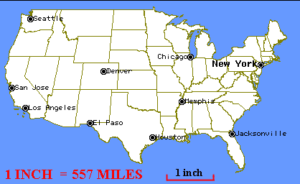## Proportions

### Learning Outcome

• Write proportions to solve proportion problemsMatryoshka or nesting dolls.

A proportion is a statement that two ratios are equal to each other. There are many things that can be represented with ratios, including the actual distance on the earth that is represented on a map. In fact, you probably use proportional reasoning on a regular basis and do not realize it. For example, say you have volunteered to provide drinks for a community event. You are asked to bring enough drinks for $35-40$ people. At the store you see that drinks come in packages of $12$. You multiply $12$ by $3$ and get $36$ – this may not be enough if $40$ people show up, so you decide to buy $4$ packages of drinks just to be sure.

This process can also be expressed as a proportional equation and solved using mathematical principles. First, we can express the number of drinks in a package as a ratio:

$\frac{12\text{ drinks }}{1\text{ package }}$

Then we express the number of people who we are buying drinks for as a ratio with the unknown number of packages we need. We will use the maximum so we have enough.

$\frac{40\text{ people }}{x\text{ packages }}$

We can find out how many packages to purchase by setting the expressions equal to each other:

$\frac{12\text{ drinks }}{1\text{ package }}=\frac{40\text{ people }}{x\text{ packages }}$

To solve for x, we can use techniques for solving linear equations, or we can cross multiply as a shortcut.

$\begin{array}{l}\,\,\,\,\,\,\,\frac{12\text{ drinks }}{1\text{ package }}=\frac{40\text{ people }}{x\text{ packages }}\\\text{}\\x\cdot\frac{12\text{ drinks }}{1\text{ package }}=\frac{40\text{ people }}{x\text{ packages }}\cdot{x}\\\text{}\\\,\,\,\,\,\,\,\,\,\,\,\,\,\,\,\,\,\,\,\,\,\,\,\,\,12x=40\\\text{}\\\,\,\,\,\,\,\,\,\,\,\,\,\,\,\,\,\,\,\,\,\,\,\,\,\,\,\,\,\,\,\,x=\frac{40}{12}=\frac{10}{3}=3.33\end{array}$

We can round up  to $4$ since it does not make sense to buy $0.33$ of a package of drinks.  Of course, you do not write out your thinking this way when you are in the grocery store, but doing so helps you to be able to apply the concepts to less obvious problems. In the following example, we will show how to use a proportion to find the number of people on the planet who do not have access to a toilet. Because, why not?

### Example

As of March, $2016$ the world’s population was estimated at $7.4$ billion. .  According to water.org, $1$ out of every $3$ people on the planet lives without access to a toilet.  Find the number of people on the planet that do not have access to a toilet.

In the next example, we will use the length of a person’s femur to estimate their height. This process is used in forensic science and anthropology. It has been found in many scientific studies to be a very good estimate.

### Example

It has been shown that a person’s height is proportional to the length of their femur . Given that a person who is $71$ inches tall has a femur length of $17.75$ inches, how tall is someone with a femur length of $16$ inches?

Another way to describe the ratio of femur length to height that we found in the last example is to say there is a $1:4$ ratio between femur length and height, or $1$ to $4$.

Ratios are also used in scale drawings. Scale drawings are enlarged or reduced drawings of objects, buildings, roads, and maps. Maps are smaller than what they represent and a drawing of dendritic cells in your brain is most likely larger than what it represents. The scale of the drawing is a ratio that represents a comparison of the length of the actual object and its representation in the drawing. The image below shows a map of the United States with a scale of $1$ inch representing $557$ miles. We could write the scale factor as a fraction $\frac{1}{557}$ or as we did with the femur-height relationship, $1:557$.A map of the United States with a scale factor.

In the next example, we will use the scale factor given in the image above to find the distance between Seattle, Washington and San Jose, California.

### Example

Given a scale factor of $1:557$ on a map of the US, if the distance from Seattle, WA to San Jose, CA is $1.5$ inches on the map,  define a proportion to find the actual distance between them.

In the next example, we will find a scale factor given the length between two cities on a map and their actual distance from each other.

### Example

Two cities are $2.5$ inches apart on a map.  Their actual distance from each other is $325$ miles.  Write a proportion to represent and solve for the scale factor for one inch of the map.

In the video that follows, we present an example of using proportions to obtain the correct amount of medication for a patient as well as finding a desired mixture of coffees.

1. "Current World Population." World Population Clock: $7.4$ Billion People $(2016)$. Accessed June $21, 2016$. http://www.worldometers.info/world-population/. "Current World Population." World Population Clock: $7.4$ Billion People $(2016)$. Accessed June $21, 2016$. http://www.worldometers.info/world-population/. "Current World Population." World Population Clock: $7.4$ Billion People $(2016)$. Accessed June $21, 2016$. http://www.worldometers.info/world-population/.
2. Obialor, Ambrose, Churchill Ihentuge, and Frank Akapuaka. "Determination of Height Using Femur Length in Adult Population of Oguta Local Government Area of Imo State Nigeria." Federation of American Societies for Experimental Biology, April $2015$. Accessed June $22, 2016$. http://www.fasebj.org/content/29/1_Supplement/LB19.short.